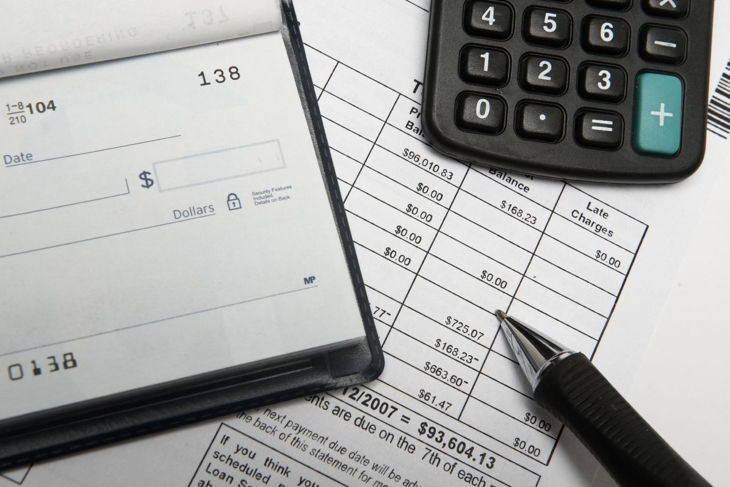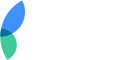With so many people having a smartphone in their pocket -- a calculator that can help determine discounts in stores, calculate a tip percentage in restaurants, or balance a checkbook in a snap -- is it really useful to be able to do fast math in your head? Many still say yes. You may not always have a calculator handy, or you may have your hands full or no cell service. Before you wonder just how useful that high school algebra class was, see if you know these best math tricks.

## Calculate Percentages in a Flash

Instead of worrying about how much to tip at a restaurant, or the dollar amount of your favorite clearance sale, use this fast trick to determine percentages. First, multiply the first number by the second number. For example, 30 percent of 50 is 30 x 50. Keep in mind that percentages are a fraction of 100, so you'll move the decimal places two points to the right. So, for 30 percent of 50, you multiply 30 x 50, which is 1500. Move your decimal point two places to the right, and you'll see that 30 percent of 50 is 15.Emanuele Cremaschi / Getty Images

Adding large numbers can be tricky. Totaling up 376 and 582 in your head may be complicated. However, if you add numbers in multiples of ten, then it becomes much easier. Simply round each number up to the nearest ten and then add. For example, round up 376 to 380 and 587 to 590. Add 380 to 590 (here's a tip: just add 38=59) and you'll have 970. Then, you just subtract the numbers that you added on to round up. in this case, 4 and 3. Then, subtract the total of those numbers from the total - 970-7. 376+582=963. Mental math is all about making the process work for you, it doesn't have to make sense to anyone else!tazytaz / Getty Images

## Divide Fairly at a Party

If you need to see if your pizza has enough slices for everyone to be served fairly, here's a quick math trick. For numbers ending in a multiple of 2, you can divide equally by 2. To divide by 3, add the digits of the number together. For instance, 501 is divisible by three because 5+0+1=6, so divisible by three. To divide into quarters, the last two digits should be divisible by four, and for five, the number should end in 5 or 0. Divisible by 9 if the digits of the entire number, added together, are divisible by nine. These simple tips should help make sure everyone gets served fairly!LauriPatterson / Getty Images

## The Rule of 72

You may be investing in your future - but for a quick estimate of how much to invest in an account that builds compound interest, use the Rule of 72. The Rule of 72 helps you determine your compound interest in an investment account and tell you how fast your money will double. Just divide the number 72 into your target interest rate, and you'll get the approximate number of years before your investment matures. for example, investing in a Certificate of Deposit (CD) at 0.9% would take 80 years for your investment to double. Consider this when planning your portfolio!acilo / Getty Images

## Remember Your 9's Times Tables

Here's a tip for the parents helping elementary kids memorize their 9s time's tables. The sum of the digits of anything multiplied by 9 equals 9. To give an example:

• 9 x 8 = 72
• 9 x 35= 315
• 9x 50 = 450

Unfortunately, that's where this trick stops, but most of these tips will work for smaller kids looking to pass the speed multiplication tests in third grade!vgajic / Getty Images

If you get paid by the hour, you can estimate what your annual salary will be, working off a 40 hour work week with no over time. Simply double your hourly rate, and you'll have a pretty close approximation as to what your annual salary would be. This information is especially valuable for those transitioning from hourly paid work to salary, as many employers ask for an annual salary in automated computer applications.Dean Mitchell / Getty Images

## Multiplying by 10 and 11

Here's another fun trick for the younger folks. When you want to multiply a number by 10, simply add a zero to the end of the number. 10 x 72 = 720. 10 x 33 = 330. want to get fancy? let your little one multiply 1,000 x 1,000. The answer? One million.

Get even fancier with the 11 multiplier trick. When multiplying by 11, put a space between the digits of the number that isn't 11. For example, 11 x 63. Then, 6_3. Add the numbers together and put the answer in the space. 6_9_3. 11 x 63 = 693!vgajic / Getty Images

## Multiplying Four by Four

This seems like it's another fun math trick for the little ones, but it can also save adults in a pinch. Many companies do accounting by the quarter - that is, a quarter of the year. If you need a quick estimate of your annual expenditures when looking at a quarterly budget, there's a simple trick to see if the line items are worth an annual investment. Simply double the number, then double it again. For example, widgets cost \$325 per quarter. Double, that's \$650 and doubled again is \$1300. Is that what you want to spend on widgets? Maybe, or maybe not but you'll have quick information at your fingertips.STEEX / Getty Images

## Fast Fractions

Sometimes fractions are tricky, especially if you're trying to pass numbers in a news article. There's a fast way to "guesstimate" fractions, even when they're awkward, like 30/50. Simply use easy benchmarks, like 1/4, 1/3, 3/4, 1/2, and so forth. then, round your fraction to the closest one and you'll have a better estimate of the size. this is especially useful when measuring cooking ingredients, or for those who track their food in volume.Jasmina81 / Getty Images

## Always Win the Number Game

Here's why you wanted to read this! Want to always win at the "pick a number" party game? Want to get the first turn for a game? Ask the group to pick a number - don't worry - with our trick, you'll always win. Then, tell them to double it. Ask them to add 6 to that sum - then tell them to divide by two. Their head is probably spinning, so tell them to subtract the number they started with. The answer? three. Just write it down on a piece of paper, and enjoy the spoils!Anchiy / Getty Images

## Popular Now on Facty

### Disclaimer

This site offers information designed for educational purposes only. The information on this Website is not intended to be comprehensive, nor does it constitute advice or our recommendation in any way. We attempt to ensure that the content is current and accurate but we do not guarantee its currency and accuracy. You should carry out your own research and/or seek your own advice before acting or relying on any of the information on this Website.Do you want to advertise on Facty.com?
Let’s talk about this! Contact us!